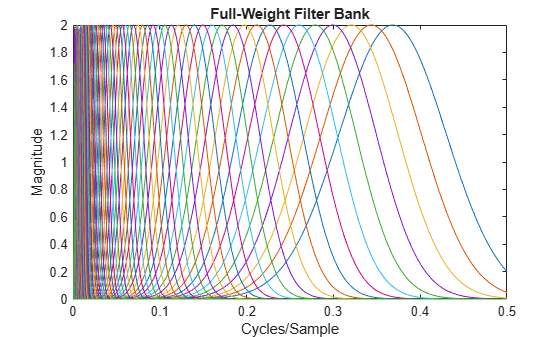# filterbank

Full-weight CWT filter bank for deep learning

## Syntax

``psif = filterbank(clayer)``

## Description

example

````psif = filterbank(clayer)` returns the full-weight continuous wavelet transform (CWT) filter bank for the `cwtLayer`, `clayer`.```

## Examples

collapse all

Create a `cwtLayer` for a signal of length 2048 samples. Specify the analytic Morlet wavelet.

`clayer = cwtLayer(SignalLength=2048,Wavelet="amor");`

Obtain the full-weight CWT filter bank of the layer.

`psif = filterbank(clayer);`

Plot the filter bank.

```slen = clayer.SignalLength; f = 0:1/slen:1-1/slen; plot(f,psif') xlim([0 1/2]) xlabel("Cycles/Sample") ylabel("Magnitude") title("Full-Weight Filter Bank")```Create a CWT filter bank. Specify the same wavelet and signal length you used to create the CWT layer. Obtain the two-sided frequency responses of the wavelet filters in the filter bank. Compare with the full-weight CWT filter bank.

```fb = cwtfilterbank(SignalLength=2048,Wavelet="amor", ... Boundary="periodic"); psidft = freqz(fb,FrequencyRange="twosided"); max(abs(psidft(:)-psif(:)))```
```ans = 9.9601e-09 ```

## Input Arguments

collapse all

CWT layer, specified as a `cwtLayer` object.

## Output Arguments

collapse all

Full-weight CWT filter bank, returned as a matrix.

Data Types: `double`

## Version History

Introduced in R2022b